# 基于时间序列分析的福建省GDP预测研究Forecast and Research on GDP of Fujian Province Based on Time Series Analysis

DOI: 10.12677/SA.2020.93046, PDF, HTML, XML, 下载: 73  浏览: 1,656

Abstract: Firstly, based on the time series analysis theory, the GDP of Fujian province from 1978 to 2015 was fitted and analyzed by Eviews software; the ARIMA(4,2,2) model and ARIMA(4,3,2) model were established. Secondly, using the two models, the GDP of Fujian province in 2016~2018 was used to test. We found the prediction error of ARIMA(4,3,2) is smaller, so it is considered that the model has a good effect. Finally using ARIMA(4,3,2) model, the GDP of Fujian province in 2019~2023 was predicted.

1. 引言

2. 数据收集Table 1. GDP of Fujian province from 1978 to 2018

3. ARIMA(4,2,2)模型的建立

3.1. 平稳性检验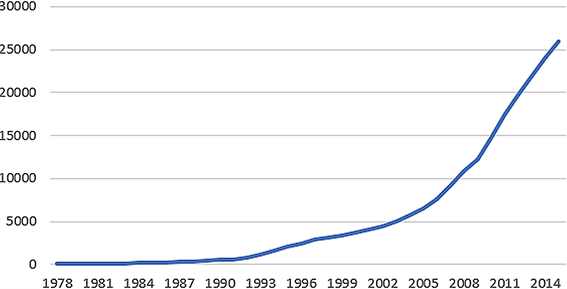Figure 1. Sequence diagram of Fujian province’s GDP from 1978~2015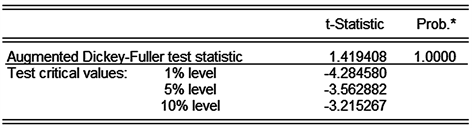Figure 2. Unit root test of {X}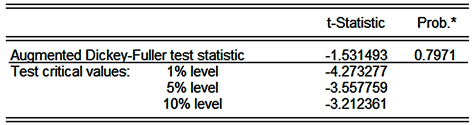Figure 3. Unit root test of {LNX}Figure 4. Unit root test of {D(LNX)}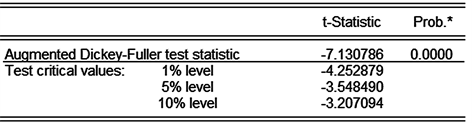Figure 5. Unit root test of {D(LNX,2)}

3.2. 模型识别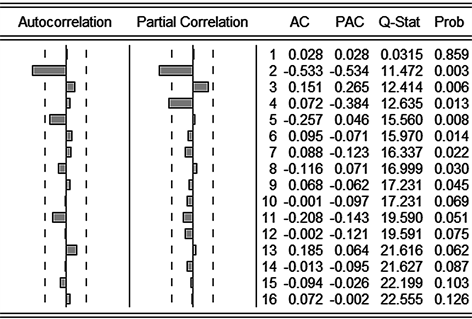Figure 6. Autocorrelation diagram and partial correlation diagram of {D(LNX,2)}

3.3. 参数估计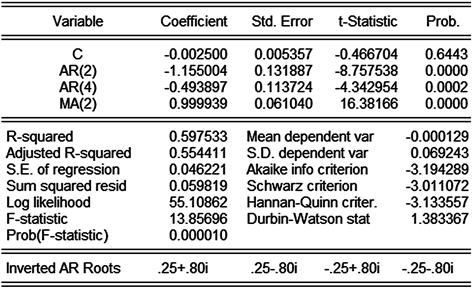Figure 7. Modeling result of ARIMA(4,2,2) after the AR(1), AR(3), MA(1) are eliminated

${x}_{t}=-0.0025-1.155004{x}_{t-2}-0.493897{x}_{t-4}+{\epsilon }_{t}-0.999939{\epsilon }_{t-2}$

3.4. 模型检验

ARIMA(4,2,2)模型残差的自相关系数均在二倍标准差内，P值也均大于0.005，所以可以认为序列之间没有任何关联，即为白噪声序列，可以用该模型进行预测。

4. ARIMA(4,3,2)模型的建立

4.1. 平稳性检验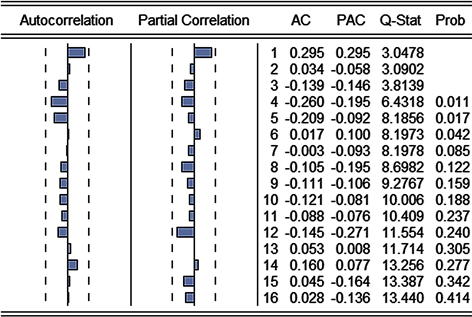Figure 8. Residual correlation diagram of ARIMA(4,2,2) model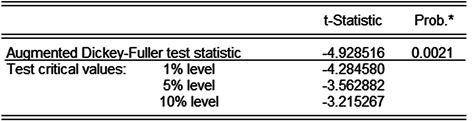Figure 9. Unit root test of {D(LNX,3)}

4.2. 模型识别

4.3. 参数估计

${x}_{t}=0.004544-1.272193{x}_{t-2}-0.605696{x}_{t-4}+{\epsilon }_{t}-0.999971{\epsilon }_{t-2}$

4.4. 模型检验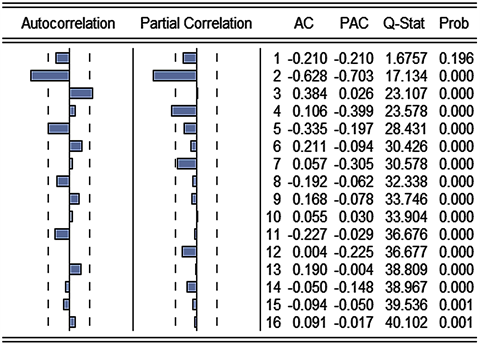Figure 10. Autocorrelation diagram and partial correlation diagram of {D(LNX,3)}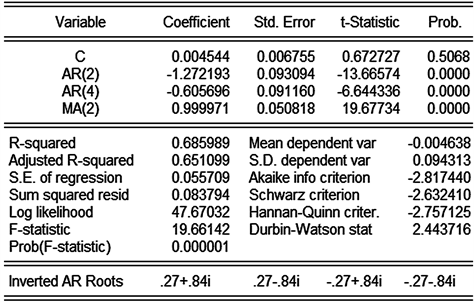Figure 11. Modeling result of ARIMA(4,3,2) after the AR(1), AR(3), MA(1) are eliminated

ARIMA(4,3,2)模型残差的自相关系数均在二倍标准差内，P值也均大于0.005，所以可以认为该模型序列之间没有任何关联，即为白噪声序列，所以可以用于预测。

5. 预测结果检验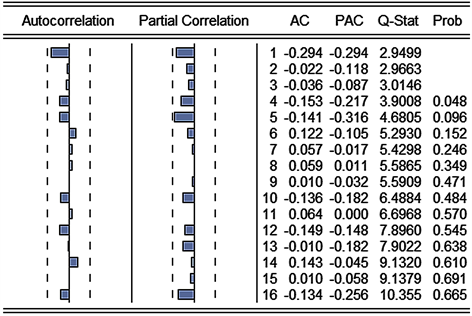Figure 12. Residual correlation diagram of ARIMA(4,3,2) modelTable 2. Comparison of model resultsTable 3. GDP forecast of Fujian province

6. 总结与展望

  宋喆磊. 改革开放以来福建省经济增长因素的实证分析[J]. 企业导报, 2009(6) : 112-113.  李守丽. 时间序列模型在地级市GDP预测中的应用[D]: [硕士学位论文]. 郑州: 郑州大学, 2013.  戴琳琳. 基于ARIMA模型的青岛市GDP预测分析[J]. 河北能源职业技术学院学报, 2019(3): 60-62.  张旭昌. 基于时间序列分析的河北省GDP预测模型研究[J]. 改革与开放, 2018(21): 16-18.  王鄂, 张霆. 时间序列在湖南省GDP预测中的应用[J]. 青岛大学学报(自然科学版), 2019, 32(3): 136-140.  孙铁轩, 邵春福. 基于ARIMA与信息粒化SVR组合模型的交通事故时序预测[J]. 清华大学学报(自然科学版), 2014, 54(3): 348-359.  福建统计局官网. 1978-2018年我国生产总值[EB/OL]. http://tjj.fujian.gov.cn/, 2020-02-01.